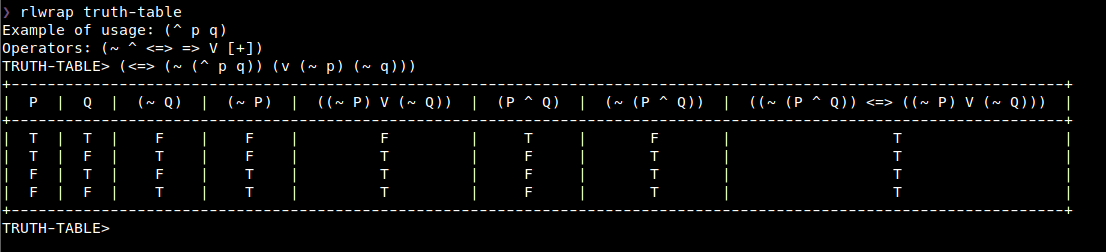#0.1

An Inference Engine using Propositional Calculus

# Lisp Inference

A non-full featured Lisp Inference Engine because I didn't implemented the reductor yet. The algorithm commonly used it's the Wang Algorithm, maybe can you help me?# Usage

The main usage for now it's using as a truth-table generator by using its command line interface. You can download the last release and use as showed in the last picture.

BSD

# Author

Manoel Vilela

## Package Index

• ### LISP-INFERENCE(INFERENCE)

• special

#### `*VALID-OPERATORS*`

No docstring provided.
• function `(`

#### `<=>`

`P Q``)`
`Biconditional binary operator`
• function `(`

#### `=>`

`P Q``)`
`Implication binary operator`
• function `(`

#### `ABSORPTION`

`EXP``)`
```Absorption inference rule ::
(=> p q) => (=> p (^ p q))```
• function `(`

#### `ADDICTION`

`EXP P``)`
```Addiction in inference rule ::
p => (v p q)```
• function `(`

#### `BICONDITIONALP`

`EXP``)`
`Verify if the expression is a biconditional`
• function `(`

#### `BINARY-OPERATIONP`

`EXP``)`
No docstring provided.
• function `(`

#### `CONJUNCTION`

`EXP P``)`
```Conjunction inference rule ::
p => (^ p q)```
• function `(`

#### `CONJUNCTIONP`

`EXP``)`
`Verify if the expression is a conjunction`
• function `(`

#### `DE-MORGAN`

`EXP``)`
```De Morgan equivalence rule ::
(~ (^ p q)) <=> (v (~ p) (~ q))
(^ p q) <=> (~ (v (~ p) (~ q)))
(~ (v p q)) <=> (^ (~ p) (~ q))
(v p q) <=> (~ (^ (~ p) (~ q)))```
• function `(`

#### `DISJUNCTIONP`

`EXP``)`
`Verify if the expression is a disjunction`
• function `(`

#### `DOUBLE-NEGATION`

`EXP``)`
```Double negation equivalence rule ::
(~ (~ p)) <=> p```
• function `(`

#### `IMPLICATIONP`

`EXP``)`
`Verify if the expression is an implication`
• function `(`

#### `INTERN-SYMBOL`

`S``)`
`Get a internal symbol reference of S`
• function `(`

#### `LOOKUP-INTERNAL-OPERATORS`

`EXP``)`
`Ensure that all operators it's inside of :lisp-inference`
• function `(`

#### `MAIN`

`)`
No docstring provided.
• function `(`

#### `MAKE-BICONDITIONAL`

`P Q``)`
No docstring provided.
• function `(`

#### `MAKE-CONJUNCTION`

`P Q``)`
No docstring provided.
• function `(`

#### `MAKE-DISJUNCTION`

`P Q``)`
No docstring provided.
• function `(`

#### `MAKE-IMPLICATION`

`P Q``)`
No docstring provided.
• function `(`

#### `MAKE-NEGATION`

`P``)`
No docstring provided.
• function `(`

#### `MODUS-PONENS`

`EXP``)`
```Modus Ponens inference rule ::
(^ (=> p q) p) => q```
• function `(`

#### `MODUS-TOLLENS`

`EXP``)`
```Modus Tollens inference rule ::
(^ (=> p q) (~ p)) => (~ q)```
• function `(`

#### `NEGATIONP`

`EXP``)`
`Verify if the expression is a negation`
• function `(`

#### `OPERATIONP`

`EXP OP``)`
```Based a 'op that can be a symbol, verify if the list
can be a operation of 'op```
• function `(`

#### `PREFIX-TO-INFIX`

`EXP``)`
No docstring provided.
• function `(`

#### `PRINT-TRUTH-TABLE`

`EXP``)`
```Given a EXP with prefixed notation generate
a pretty truth-table for each grouped case.```
• function `(`

#### `PROPOSITIONP`

`SYMBOL``)`
`Check if the given SYMBOL can be a proposition (letters)`
• function `(`

#### `SIMPLIFICATION-FIRST`

`EXP``)`
```Simplification with first operand rule ::
(^ p q) => p```
• function `(`

#### `SIMPLIFICATION-SECOND`

`EXP``)`
```Simplification with second operand rule ::
(^ p q) => q```
• function `(`

#### `SYLLOGISM-DISJUNCTIVE`

`EXP``)`
```Syllogism Disjunctive inference rule ::
(^ (v p q) (~ p)) => q```
• function `(`

#### `SYLLOGISM-HYPOTHETICAL`

`EXP``)`
```Syllogism Hypothetical inference rule ::
(^ (=> x y) (=> y z)) => (=> x z)
(^ (=> y z) (=> x y)) => (=> x z)```
• function `(`

#### `TESTS`

`)`
No docstring provided.
• function `(`

#### `UNARY-OPERATIONP`

`EXP``)`
No docstring provided.
• function `(`

#### `V`

`P Q``)`
`Disjunction binary operator`
• function `(`

#### `VALID-OPERATIONP`

`EXP``)`
No docstring provided.
• function `(`

#### `[+]`

`P Q``)`
`XOR operator or exclusive disjunction operator`
• function `(`

#### `^`

`P Q``)`
`Conjuction binary operator`
• function `(`

#### `~`

`P``)`
`Not unary operator`
• macro `(`

#### `TRUTH`

`EXP``)`
`A easy way to generate a truth table`
• macro `(`

#### `TRUTH-INFIX`

`EXP``)`
```A easy and infix way of EXP generate a truth table.
Ex.: (truth-infix (p ^ q)) ```